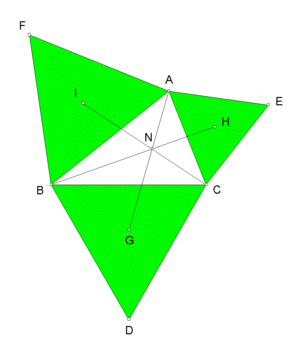# NAPOLEON POINTS

 Suppose ABC is a triangle. Let points D,E,F be points, as in the figure, for which the three triangles DBC, CAE, ABF are equilateral. Let G = center of triangle DBC, H = center of triangle CAE, I = center of triangle ABF The lines AG, BH, CI meet in a point. Labeled N, it is called the first Napoleon point. Trilinear coordinates for N are csc(A + π/6): csc(B + π/6): csc(C + π/6).If the three equilateral triangles point inward instead of away from triangle ABC, the three lines AG, BH, CI meet in the second Napoleon point, with trilinears csc(A - π/6): csc(B - π/6): csc(C - π/6).

References:

John Rigby, "Napoleon revisited," Journal of Geometry 33 (1988) 129-146.